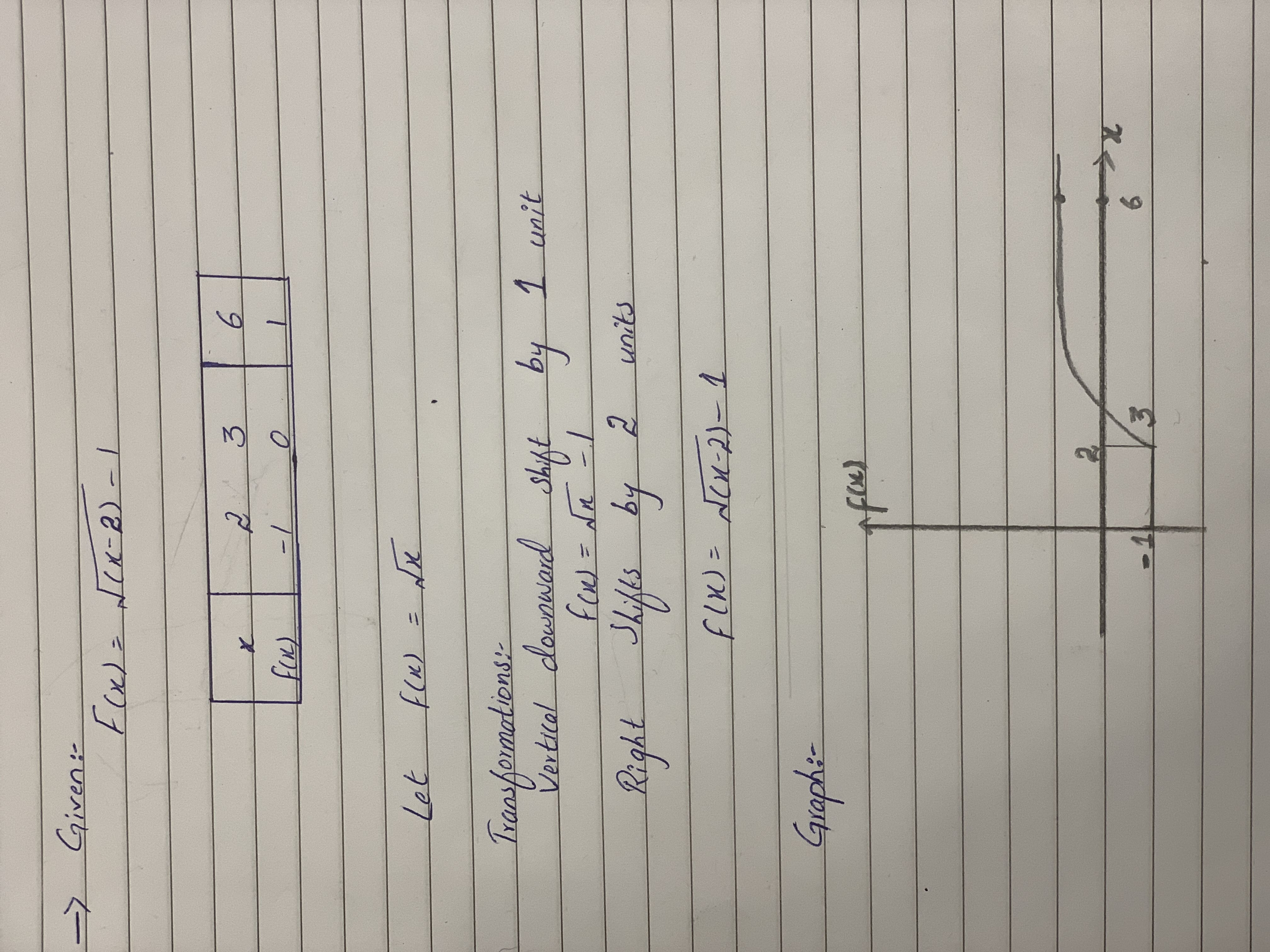Question# Graph by labeling three points and determine the type or types of transformations:h(x)=sqrt(x-2)-1

Performing transformations
ANSWEREDGraph by labeling three points and determine the type or types of transformations:
$$h(x)=\sqrt{x-2}-1$$